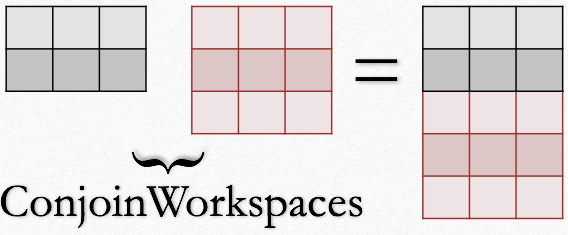$$\renewcommand\AA{\unicode{x212B}}$$

# ConjoinWorkspaces v1¶

## Summary¶

Join two workspaces together by appending their spectra.

## Properties¶

Name

Direction

Type

Default

Description

InputWorkspace1

InOut

MatrixWorkspace

Mandatory

The name of the first input workspace

InputWorkspace2

Input

MatrixWorkspace

Mandatory

The name of the second input workspace

CheckOverlapping

Input

boolean

True

Verify that the supplied data do not overlap

YAxisLabel

Input

string

The label to set the Y axis to

YAxisUnit

Input

string

The unit to set the Y axis to

## Description¶

This algorithm can be useful when working with large datasets. It enables the raw file to be loaded in two parts (not necessarily of equal size), the data processed in turn and the results joined back together into a single dataset. This can help avoid memory problems either because intermediate workspaces will be smaller and/or because the data will be much reduced after processing.

The output of the algorithm, in which the data from the second input workspace will be appended to the first, will be stored under the name of the first input workspace. Workspace data members other than the data (e.g. instrument etc.) will be copied from the first input workspace (but if they’re not identical anyway, then you probably shouldn’t be using this algorithm!). Both input workspaces will be deleted.

## ConjoinWorkspaces operation¶

 Example case with input workspaces having 2 and 3 spectra respectively.### Conflict Spectrum Numbers¶

The algorithm adds the spectra from the first workspace and then the second workspace.

• The original spectrum Nos will be respected if there is no conflict of spectrum Nos between the first workspace and the second.

• If there are conflict in spectrum Nos, such that some spectrum Nos appear in both workspace1 and workspace2, then it will be resolved such that the spectrum Nos of spectra coming from workspace2 will be reset to some integer numbers larger than the largest spectrum No of the spectra from workspace1. Assuming that the largest spectrum No of workspace1 is S, then for any spectrum of workspace wi in workspace2, its spectrum No is equal to (S+1)+wi+offset, where offset is a non-negative integer.

### Restrictions on the input workspace¶

The input workspaces must come from the same instrument, have common units and bins and no detectors that contribute to spectra should overlap.

### Y axis units and labels¶

The optional parameters YAxisUnit and YAxisLabel can be used to change the y axis unit and label when conjoining workspaces. Changing YAxisUnit updates YAxisLabel automatically with the value of YAxisUnit, unless a separate value is supplied.

### Exception¶

If property ‘CheckOverlapping’ is set to true, and there are spectra and/or detectors are overlapping between two input workspaces, then an ‘invalid_argument’ exception will be thrown from function ‘CheckForOverlap’.

## Usage¶

ConjoinWorkspaces Example

ws1 = CreateSampleWorkspace(WorkspaceType="Histogram", NumBanks=2, BankPixelWidth=1, BinWidth=10, Xmax=50)
print("Number of spectra in first workspace = {}".format(ws1.getNumberHistograms()))
ws2 = CreateSampleWorkspace(WorkspaceType="Histogram", NumBanks=3, BankPixelWidth=1, BinWidth=10, Xmax=50)
print("Number of spectra in second workspace = {}".format(ws2.getNumberHistograms()))
ConjoinWorkspaces(InputWorkspace1=ws1, InputWorkspace2=ws2, CheckOverlapping=False, YAxisUnit="New unit", YAxisLabel="New label")
ws = mtd['ws1'] # Have to update workspace from ADS, as it is an in-out parameter
print("Number of spectra after ConjoinWorkspaces = {}".format(ws.getNumberHistograms()))
print("Y unit is {}".format(ws.YUnit()))
print("Y label {}".format(ws.YUnitLabel()))


Output:

Number of spectra in first workspace = 2
Number of spectra in second workspace = 3
Number of spectra after ConjoinWorkspaces = 5
Y unit is New unit
Y label New label


Categories: AlgorithmIndex | Transforms\Merging